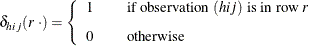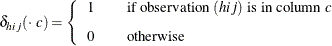# The SURVEYFREQ Procedure

#### Definitions and Notation

For a stratified clustered sample design, define the following: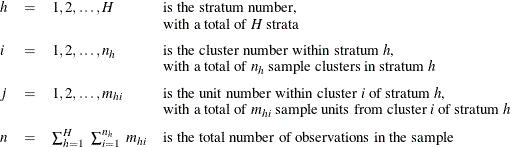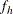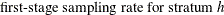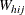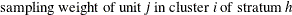The sampling rate, which is used in Taylor series variance estimation, is the fraction of first-stage units (PSUs) selected for the sample. You can specify the stratum sampling rates with the RATE= option. Or if you specify population totals with the TOTAL= option, PROC SURVEYFREQ computesas the ratio of stratum sample size to the stratum total, in terms of PSUs. See the section Population Totals and Sampling Rates for details. If you do not specify the RATE= option or the TOTAL= option, then the procedure assumes that the stratum sampling ratesare negligible and does not use a finite population correction when computing variances.

This notation is also applicable to other sample designs. For example, for a design without stratification, you can let H = 1; for a sample design without clustering, you can let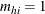for every h and i, which replaces clusters with individual sampling units.

For a two-way table representing the crosstabulation of two variables, define the following, where there are R levels of the row variable and C levels of the column variable: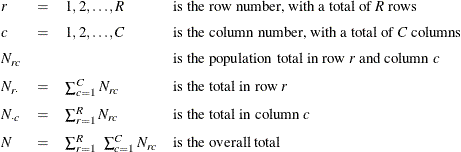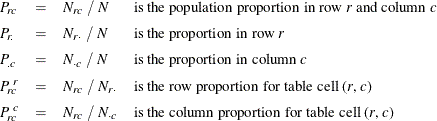For a specified observation (identified by stratum, cluster, and unit number within the cluster), define the following to indicate whether or not that observation belongs to cell (r, c), row r and column c, of the two-way table, for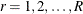and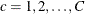: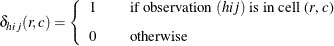Similarly, define the following functions to indicate the observation’s row and column classification: